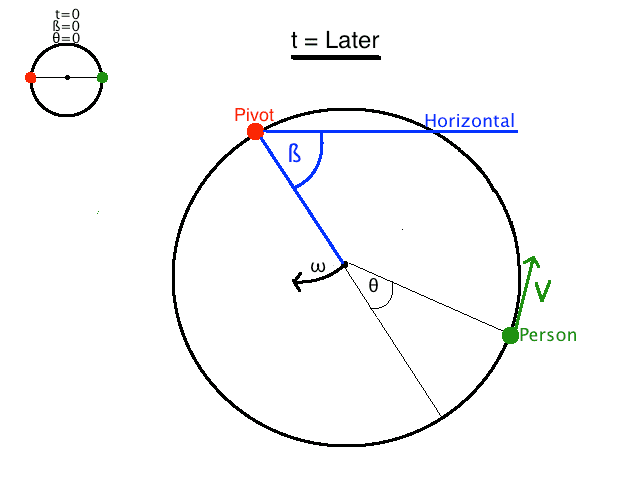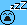# Person walking on the circumference of a disc

• Nathanael

Homework Helper

## Homework Statement

A man is walking at a constant velocity v along the circumference of a disc which is pivoted at a point on it's circumference. The man starts diametrically opposite to the pivot and the disc starts at rest. The man and disc have the same mass. When the man reaches back to his original place on the disc, what will the angular displacement of the disc be?

2. Relevant stuff
I've made a picture to illustrate the problem and (mostly) to illustrate my attempt at a solution. The top left corner of the picture shows the system at time zero, and the bigger part of the picture shows the system at some arbitrary later time (when the man has walked a distance θ, so I guess the time would be T=θR/v, but that's not important).

## The Attempt at a Solution

ß is the angular displacement of the disc (as shown in the picture)
θ is the angular displacement of the man relative to the disc (as shown in the picture)
ω is the angular velocity of the disc with respect to the pivot
Let's define L to be the distance between the pivot and the person (which depends on θ)
And for just a moment let's say L is a vector from the pivot to the person, then let's define V as the component of the person's velocity which is perpendicular to LFrom conservation of angular momentum:
$mV_⊥L=Iω$
$I$, the moment of inertia of the disc, should be $\frac{3}{2}mR^2$ so we get:
$V_⊥=\frac{3ωR^2}{2L}$

We can determine the radial component of the velocity Vr from $V_⊥^2+V_r^2=V^2$

I think the last constraint is that the direction of the velocity is tangent to the circle. From geometric considerations I get:
$\tan(\frac{θ}{2})=\frac{V_r}{V_⊥}$

Also I forgot to mention $L=\sqrt{2(1+cos(θ)}$

So from all this you get ω as a function of θ, but since v is constant, $θ=\frac{vt}{R}$ and so we have ω(t)
Since $β=∫_0^Tω(t)dt$ the answer should be $∫_0^{\frac{2\pi R}{v}}ω(t)dt$ but this does not give me the correct answer.

•arpon and vktsn0303
I just realized, as I was re-reading my OP, that I think the conservation of angular momentum equation may be something like $mL(V_⊥-ωL)=Iω$
I'll work it out in the morning with this equation and see where it gets me, but any help is still appreciated.

Of course, I couldn't wait to the morning (who wants to sleep with a potentially solved problem on their mind).
But I did cheat and use an integral calculator (it was too ugly!)

It turns out that both equations for conservation of angular momentum (from post #1 and #2) give an answer which depends on R the radius of disc.

I must have made a mistake, because the answer is supposed to be independent of R

$L=\sqrt{2(1+cos(θ)}$
I have no idea how I realized this mistake while virtually asleep... but this is just a typo. It is supposed to be $L=R\sqrt{2(1+cos(θ)}$

BUT I'm pretty sure the expression I integrated (with a calculator) inherited this typo, which explains why the answer depended on R
I probably still made a mistake somewhere in my logic... but this time I really will wait until morning to check the answerGood morning ! You have a nice touch in finding challenging questions! I don't have any solutions, no expertise, no bright ideas and little time to contribute. But will be watching this thread with interest.

Do want to share the first question that comes to mind: Your initial situation has angular momentum zero, I should think (from the way you wrote angular momentum conservation). No external torque, so it stays zero. Your expressions are zero when L is zero. Feels contra-intuitive, but can't be excluded (after all, maintaining a constant v in a rotating frame of reference has consequences that are hard to imagine). When the guy is back on the spot on the disc where he started, should the angular momentum be zero again ?

Do you really evaluate ##V_\perp## in the fixed frame of reference ? I don't get that impression.

I think I understand your drawing. The term Horizontal is confusing, though.

constant velocity v along the circumference of a disc
I guess you ,mean constant speed;)
the expression I integrated
Would you mind posting the integral you get?
When the guy is back on the spot on the disc where he started, should the angular momentum be zero again ?
The angular momentum of the system about the pivot point will be zero throughout. I feel you meant something else.

I think I understand your drawing. The term Horizontal is confusing, though.
Right, I did not realize how that could be confusing but now it's painfully obvious... indeed the entire problem takes place in a horizontal plane, sorry! I just meant to show that I was measuring the angular displacement of the disc ß from the starting position (sorry for the possible confusion).
Do you really evaluate ##V_\perp## in the fixed frame of reference ? I don't get that impression.
I'm not exactly sure what you're asking. If you're asking about my personal problem solving method then yes, my mind is in the fixed frame of reference. I always solve problems in inertial frames; I'm uncomfortable with non-inertial frames.
Your initial situation has angular momentum zero, I should think (from the way you wrote angular momentum conservation).
Yes the system should have zero angular momentum. I imagine the person standing on the disc and everything is still, and then when time zero comes the person begins walking. No matter how odd or complex the path of the person is, the angular momentum should always be zero.
Your expressions are zero when L is zero.
When L=0 the person is walking on the pivot. The only possibility which conserves momentum would be for the disc to have zero angular velocity at that instant.
I guess you ,mean constant speed;)
:)
Would you mind posting the integral you get?

$\int_0^{\frac{2piR}{v}}\frac{v\cos(\frac{vt}{2r})}{L+\frac{3R^2}{2L}}dt$

Where $L=R\sqrt{2(1+\cos(\theta))}$ (I didn't want to plug it in and make too messy)

Perhaps I should explain where it comes from.

The conservation of angular momentum equation in post#2 gives me $V_⊥=ω(L+\frac{3R^2}{2L})$

From post#1 I have:

$\tan(\frac{θ}{2})=\frac{V_r}{V_⊥}=\frac{\sqrt{v^2-V_⊥^2}}{V_⊥}$

$\tan^2(\frac{θ}{2})=\frac{v^2-V_⊥^2}{V_⊥^2}=\frac{v^2}{V_⊥^2}-1$

$\frac{v^2}{V_⊥^2}=\tan^2(\frac{θ}{2})+1=\sec^2(\frac{\theta}{2})$

$V_⊥=v\cos(\frac{\theta}{2})=ω(L+\frac{3R^2}{2L})$

And from that we get ω(θ) then $θ=\frac{vt}{R}$ takes you to my integral

Last edited:
$\int_0^{\frac{2piR}{v}}\frac{v\cos(\frac{vt}{2r})}{L+\frac{3R^2}{2L}}dt$

Where $L=R\sqrt{2(1+\cos(\theta))}$
I get the same, but in a very much simpler form!
Try using ##1+\cos(\theta) = 2 \cos^2(\theta/2)## etc.
It comes down to the form ##\frac 12 \int_0^{2\pi}\frac{1+\cos(\theta)}{A+\cos(\theta)}.d\theta##, some constant A. Still a painful integral, though.

•Nathanael
Try using ##1+\cos(\theta) = 2 \cos^2(\theta/2)## etc.
Thanks, I'm really bad with trig identities. Then $L=2R\cos(\frac{\theta}{2})$
So then the integral becomes $\int_0^T\frac{4v\cos^2(\frac{\theta}{2})}{8R\cos^2(\frac{\theta}{2})+3R}dt$

I suppose the smart thing to do (instead of replacing $\theta=\frac{vt}{R}$) would be to replace $dt=\frac{R}{v}d\theta$ which gives

$\int_0^{2\pi}\frac{4\cos^2(\frac{\theta}{2})}{8\cos^2(\frac{\theta}{2})+3}d\theta$

Intuitively it's clear, but I'm not exactly sure, mathematically, why T becomes 2π. I guess since we multiplied the function by R/v then we must multiply the limits by v/R? (So that the endpoints of the integral are unchanged?) So then $T=\frac{2\pi R}{v}$ becomes $2\pi$

I guess to further simplify it you used $\cos^2(\frac{\theta}{2})=\frac{1}{2}(1+\cos\theta)$ which turns it into:

$\frac{1}{2}\int_0^{2\pi}\frac{1+\cos\theta}{\frac{7}{4}+\cos\theta}d\theta$

Which I think can be simplified a bit more into the form

$\frac{1}{2}\int_0^{2\pi}(1-\frac{3}{7+4\cos\theta})d\theta=\pi-\frac{1}{2}\int_0^{2\pi}\frac{3}{7+4\cos\theta}d\theta$

Last edited:
$\int_0^{2\pi}\frac{4\cos^2(\frac{\theta}{2})}{8\cos^2(\frac{\theta}{2})+3}d\theta$

Intuitively it's clear, but I'm not exactly sure, mathematically, why T becomes 2π. I guess since we multiplied the function by R/v then we must multiply the limits by v/R? (So that the endpoints of the integral are unchanged?) So then $T=\frac{2\pi R}{v}$ becomes $2\pi$

You can change the integrating variable from t to theta, using the relation θR=Vt. dt=R/V dθ, and the limits also change: the upper limit is θ(T) which is 2pi, as the person gets back to its initial position on the disk, so its angular displacement is 2pi.

As for the integral, a useful substitution is u=tan(θ/2), dθ=2/(1+u2) du. You get a rational function to integrate.

•Nathanael
$\pi-\frac{1}{2}\int_0^{2\pi}\frac{3}{7+4\cos\theta}d\theta$
I haven't been able to figure out a method for this integral, so I cheated out of curiosityand looked it up, and the answer is indeed correct! :)

•